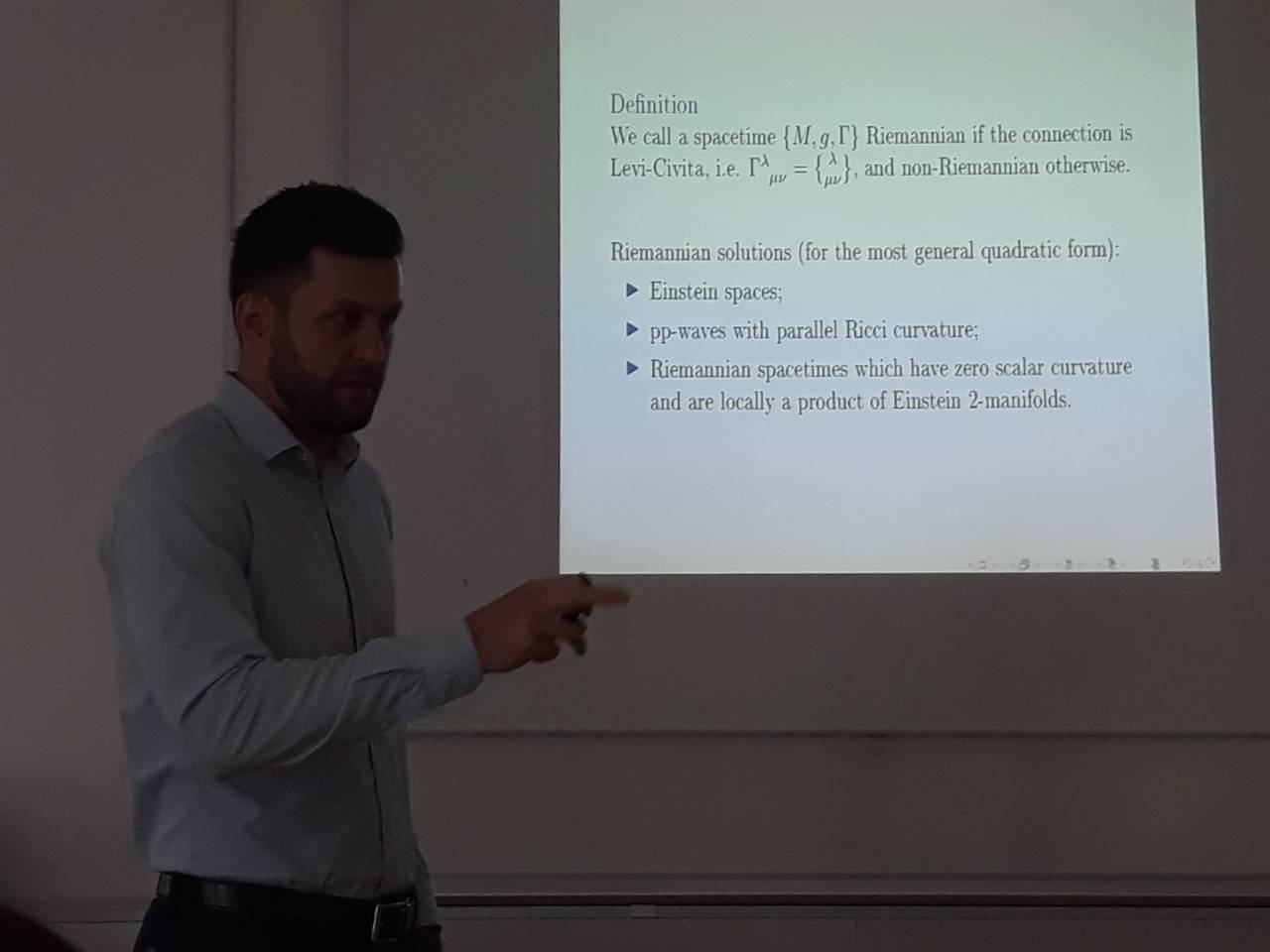Univerza na Primorskem Fakulteta za matematiko, naravoslovje in informacijske tehnologije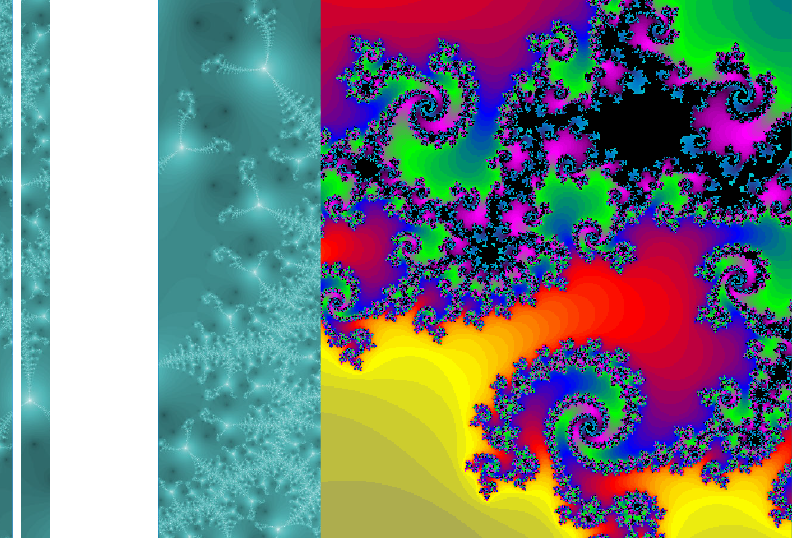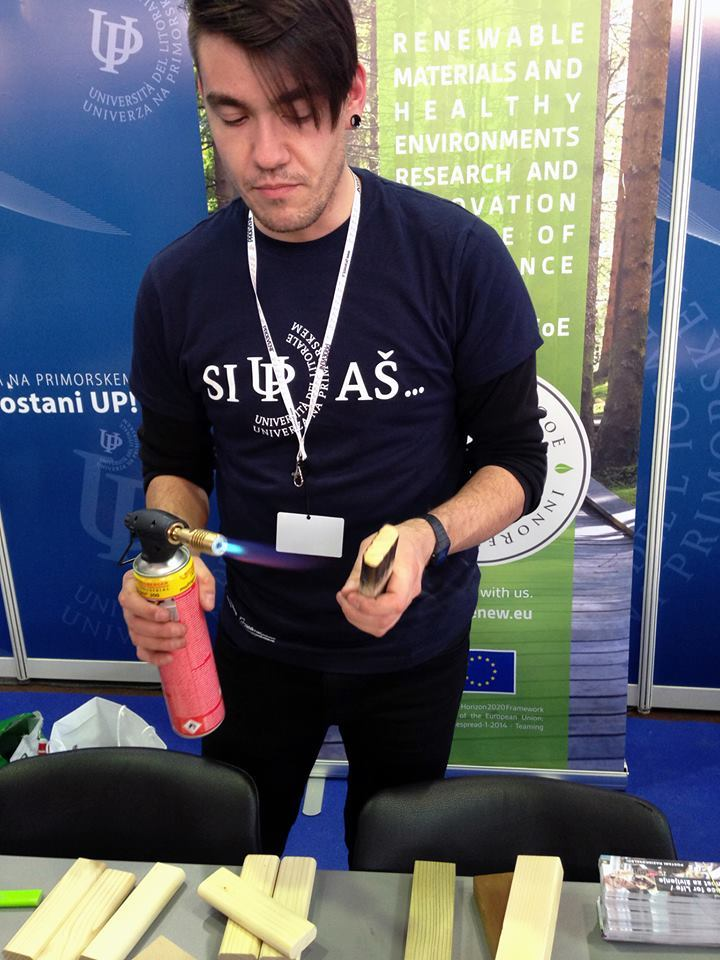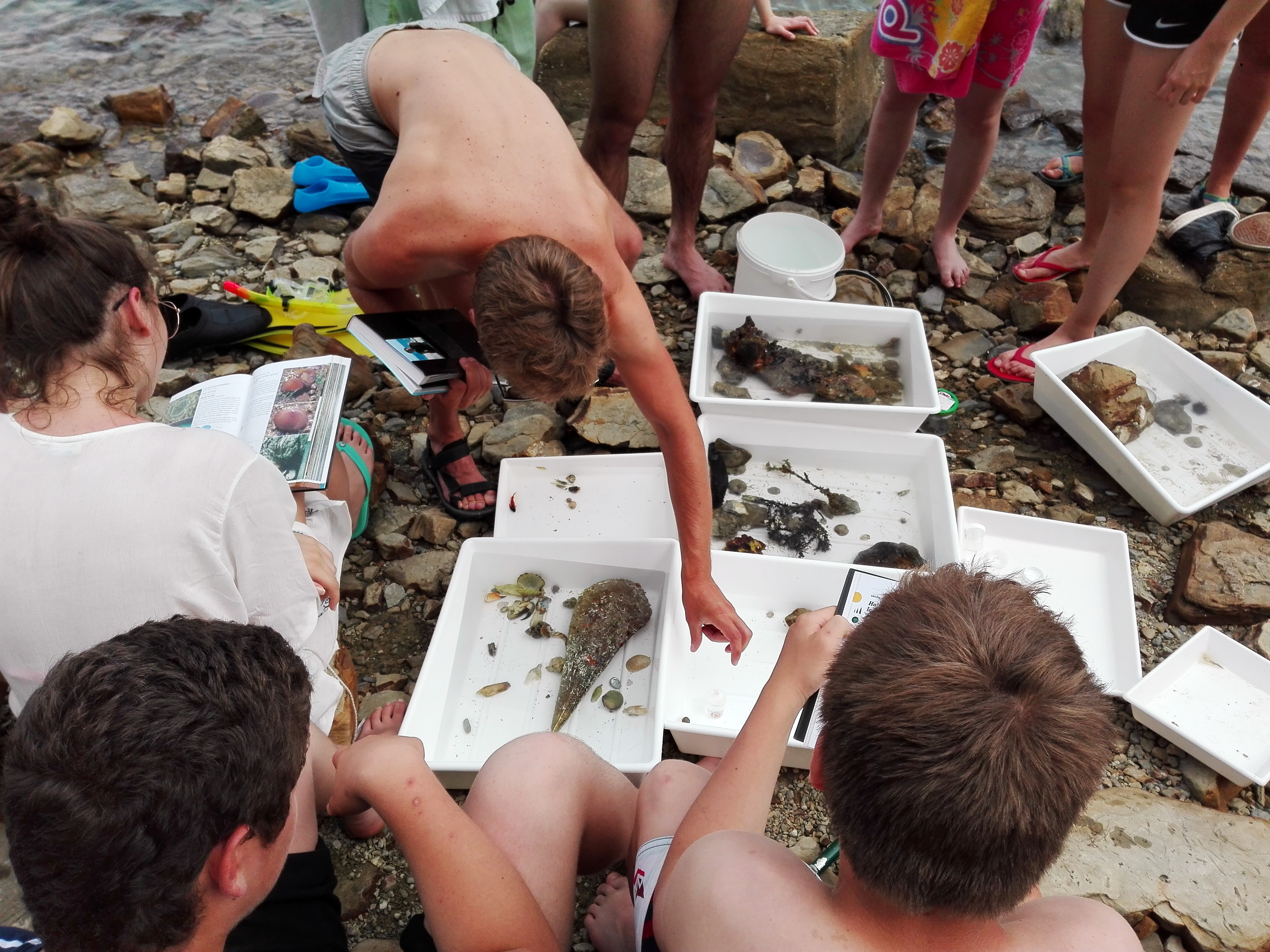SI | EN# Raziskovalni matematični seminar - Arhiv

 2022 2021 2020 2019 2018 2017 2016 2015 2014 2013 2012 2011 2010
 1 2 3 4 5 6 7 8 9 10 11 12
Datum in ura / Date and time: 3.6.19
(10:00--11:00)
Predavalnica / Location: FAMNIT-MP1
Predavatelj / Lecturer: Žiga Velkavrh (University of Primorska)
Naslov / Title: Reputation and (Dis)honesty: A game-theoretic approach
Vsebina / Abstract:

In the first part of this talk we introduce Game Theory, its solution concepts and applications. We then discuss the limits of classical game-theoretic predictions and give possible reasons for that. At the end of the first part we present some behavioral and evolutionary models. These models have better predictive power than classical models and are therefore more useful in real life.

In the second part of this talk a joint work with Assoc. Prof. A. Ule is presented. The main topics of our research are reputation and (dis)honesty.  Dishonesty has a negative impact on everyone if and when the truth is revealed, and the consequences can be severe. How dishonesty spreads through the social network? Can we eliminate (or at least reduce) harmful lies and mistrust, and thus inefficiency? We try to answer these kind of questions.

We are also interesting in finding stable strategies, i.e. strategies that persist over time. We present our theoretical model and (partial) results. In order to get some new insights about people behavior (e.g. what strategies do people use), we also ran a pilot experiment. The experimental results are presented in the last part of the talk.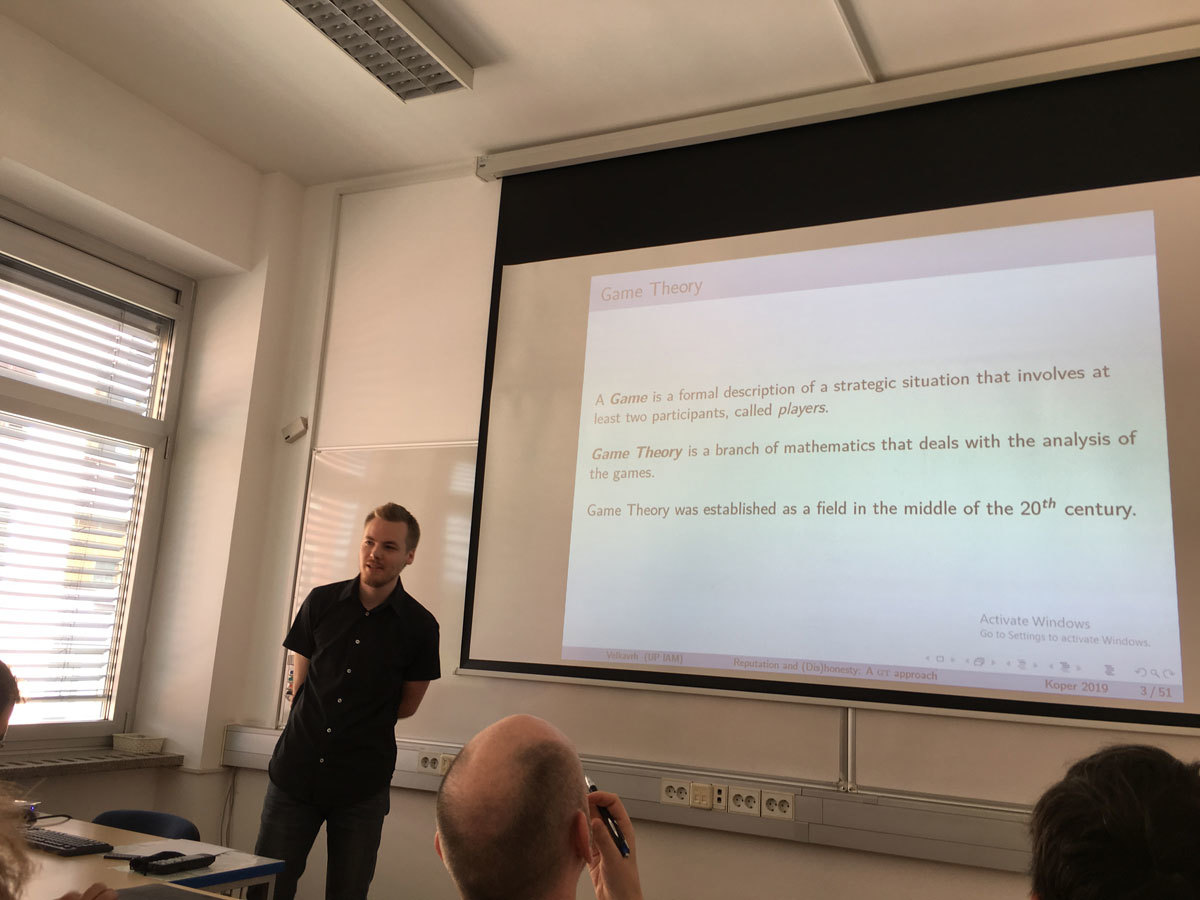Datum in ura / Date and time: 27.5.19
(10:00--11:00)
Predavalnica / Location: FAMNIT-MP1
Predavatelj / Lecturer: Tibor Illés (Budapest University of Technology and Economics, Institute of Mathematics, Hungary)
Naslov / Title: Sufficient linear complementarity problems: matrix classes, algorithms and applications
Vsebina / Abstract:

Linear complementarity problems (LCP) generalizes some fundamental problems of mathematical optimization like linear programming (LP) problem, linearly constrained quadratic programming (LQP) problem and some other. It admits an enormous number of applications in economics, engineering, science and many other fields. After all these, it is not surprising that LCPs are usually NP-complete problems (S.J. Chung, 1989).

The three most significant classes of algorithms for solving LCP problems are: pivot algorithms (PA), interior point algorithms (IPA) and continuation methods. Because, both PA and IPA have been developed earlier for LP and QP problems it is quite natural idea to test them on LCP problems, as well.

Concept of sufficient matrices, as generalization of positive semidefinite matrices, has been introduced 30 years ago by Cottle et al. (1989). LCPs with sufficient matrices posses many important properties, like the solution set is convex and polyhedral; guarantees the finiteness of PAs and (pseudo) polynomial behaviour of the IPAs.

The largest matrix class where the interior point algorithms (IPA) are polynomial is the class of P*(κ)-matrices, for given nonnegative real parameter κ. The union for all possible κ parameters of P*(κ)-matrices forms the class of P*-matrices. This class of matrices has been introduced by Kojima et al. in 1991. Known IPAs for LCPs with P*(κ)-matrices under the interior point assumption, possess polynomial iteration complexity depending on the problem size n, parameter κ and the bit length L of the rational data of the LCP.

After all of these, it is a natural question: What is the relation between the sufficient and P*-matrices? Väliaho (1996) proved that the P*-matrices are exactly those which are sufficient. Unfortunately, there are two important, negative results related to sufficient matrices. P. Tseng (2000) proved that decision problem related to the membership of matrices for P0- and column sufficient matrices are all co-NP-complete. While de Klerk and Eisenberg-Nagy showed that there exists such P*(κ)-matrices for which the κ value is exponential in the size n of the problem.

Furthermore, for sufficient LCPs, it is meaningful to introduce dual LCP problem and it can be proved that from sufficient primal- and dual LCP problem, exactly one has solution, that is an interesting, nice and (quite) new generalization of the old Farkas’ lemma.

There are still several open questions in the area of sufficient LCPs. More importantly, solution methods developed for sufficient LCPs helps us in trying to solve LCP problems with more general matrices.

e-mail: illes@math.bme.hu
homepage: https://det.math.bme.hu/illes-tibor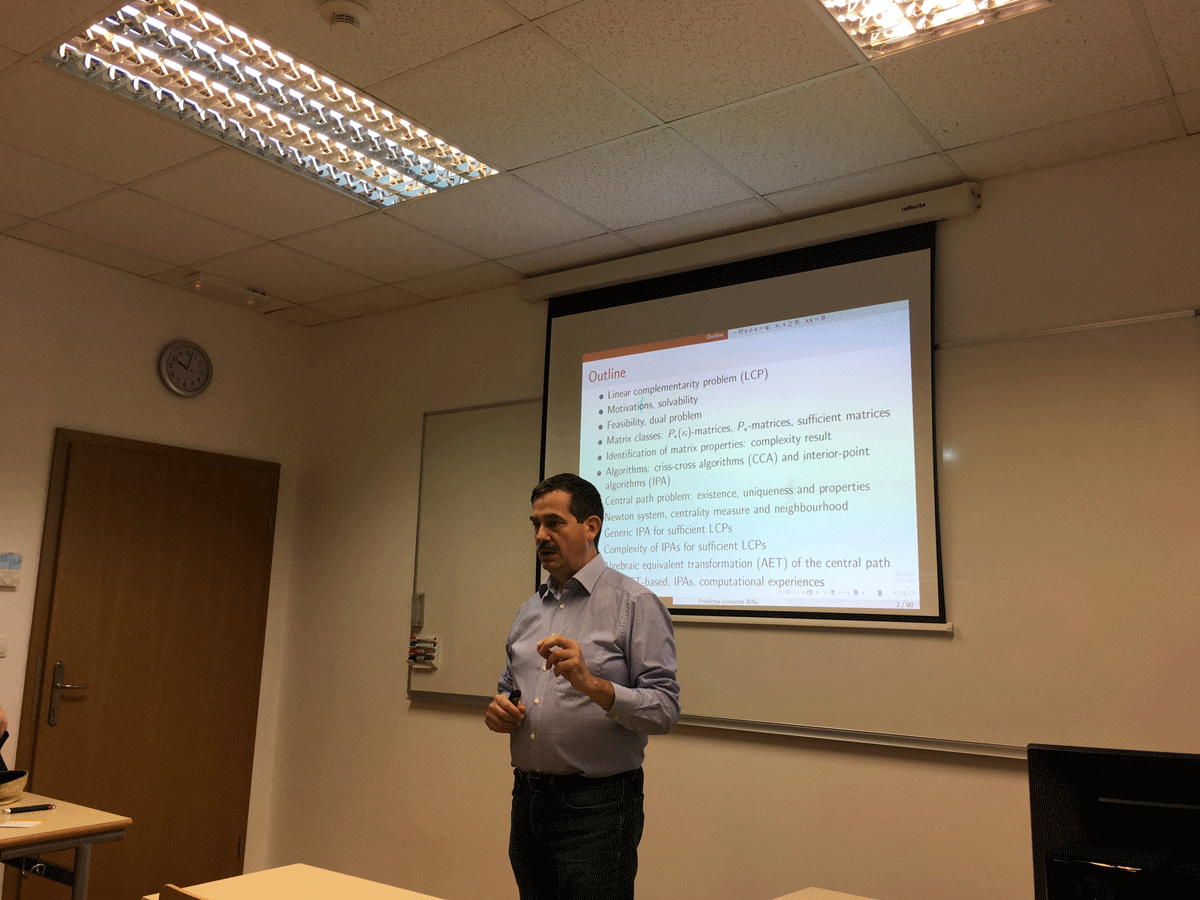Datum in ura / Date and time: 20.5.19
(10:00--11:00)
Predavalnica / Location: FAMNIT-MP1
Predavatelj / Lecturer: Daniel Hawtin (University of Rijeka, Croatia)
Naslov / Title: Binary Completely Transitive Codes
Vsebina / Abstract:

Given a code in a graph, the vertex set of the graph may be partitioned by the sets of vertices at each of the possible distances to the code. If the automorphism group of the code acts transitively on each cell of this `distance partition', then the code is called `completely transitive'. I will discuss recent work towards a classification of binary completely transitive codes, and briefly discuss related algebraic and combinatorial symmetry conditions.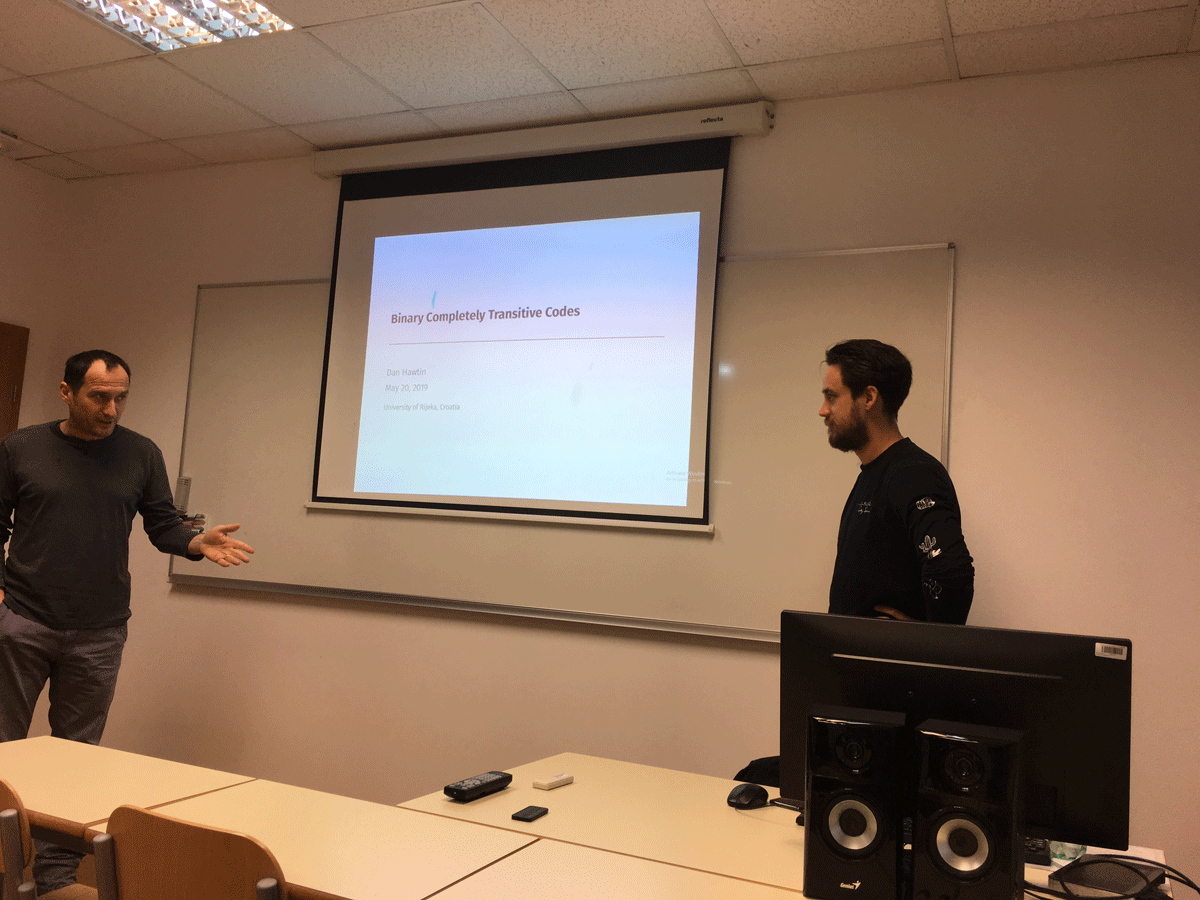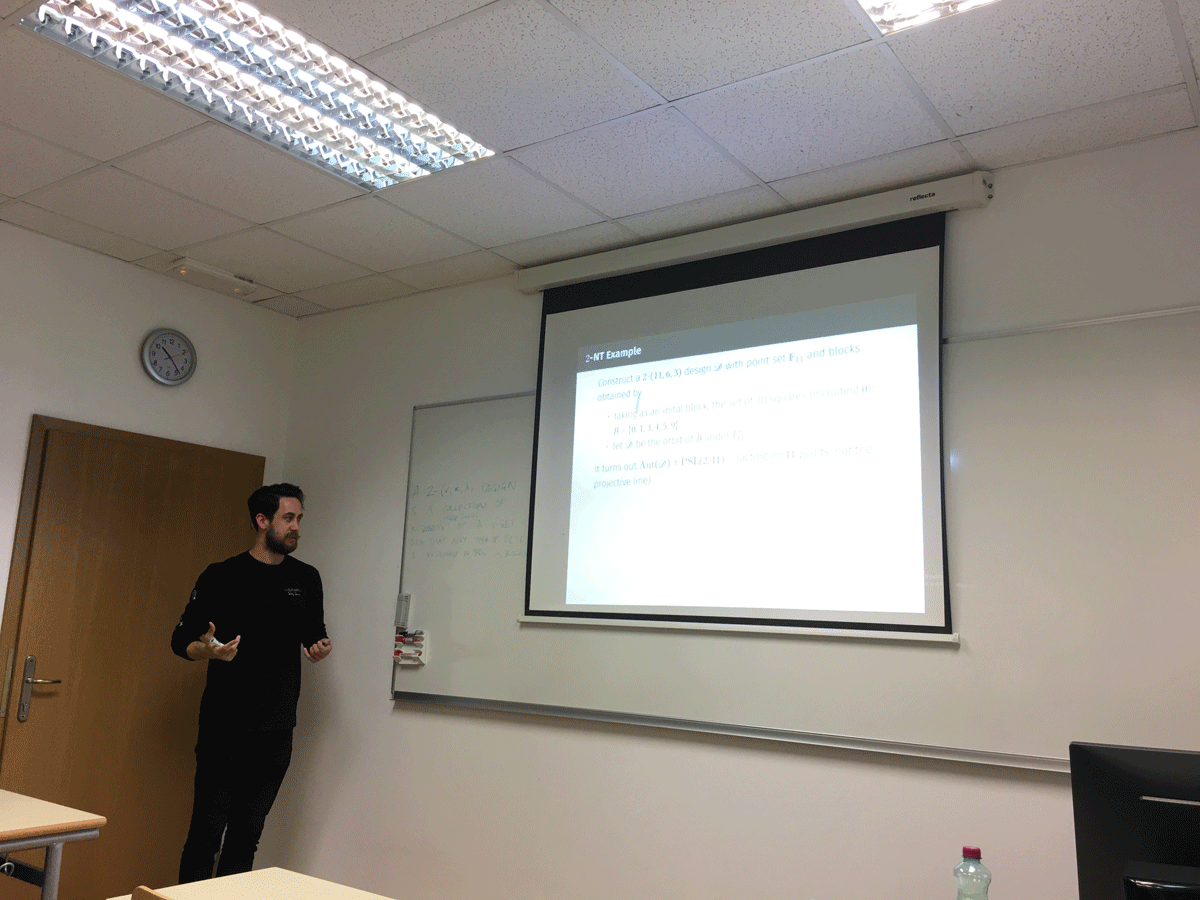Datum in ura / Date and time: 13.5.19
(10:00--11:00)
Predavalnica / Location: FAMNIT-MP1
Predavatelj / Lecturer: Nina Chiarelli (FAMNIT and IAM, UP)
Naslov / Title: Linear separation of connected dominating sets in graphs
Vsebina / Abstract:

A connected dominating set in a graph is a dominating set of vertices that induces a connected subgraph. In this talk we present graphs in which connected dominating sets can be separated from the other vertex subsets by a linear weight function. More precisely, we say that a graph is connected-domishold if it admits non-negative real weights associated to its vertices such that a set of vertices is a connected dominating set if and only if the sum of the corresponding weights exceeds a certain threshold. We characterize the graphs in this non-hereditary class in terms of a property of the set of minimal cutsets of the graph, and we also give several characterizations for the hereditary case, that is, when each connected induced subgraph is required to be connected-domishold.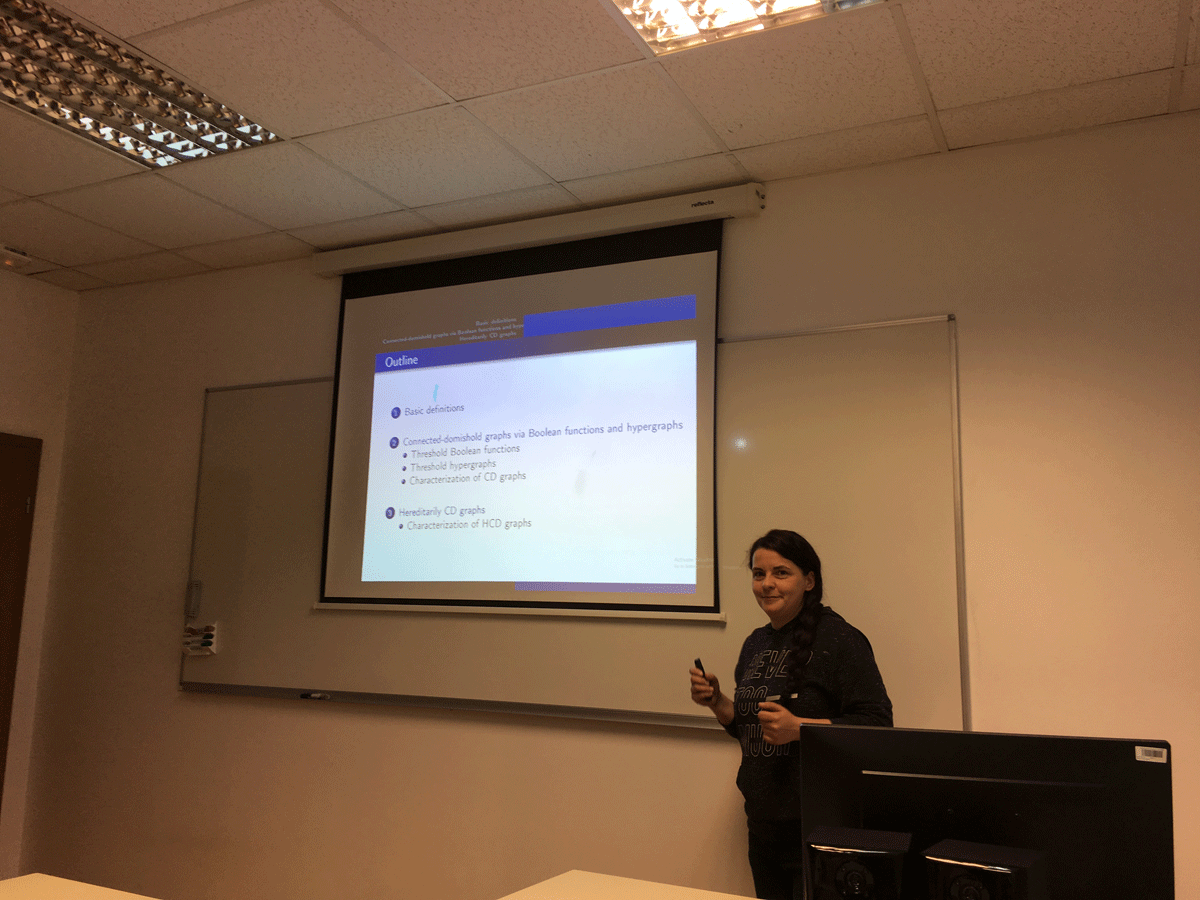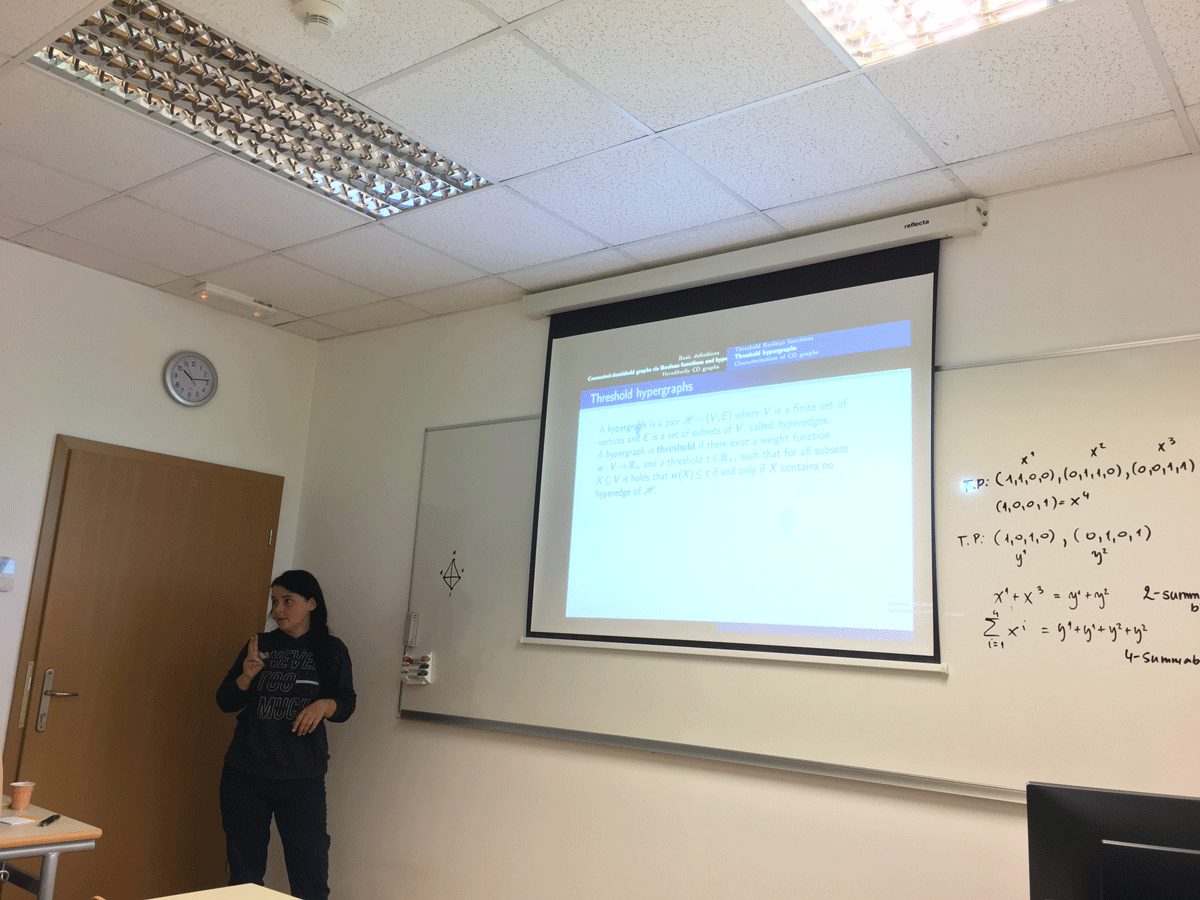Datum in ura / Date and time: 16.5.19
(11:00--12:00)
Predavalnica / Location: FAMNIT-VP2
Predavatelj / Lecturer: Elvis Baraković (University of Tuzla, Bosnia and Herzegovina)
Naslov / Title: Axial torsion waves in metric-affine gravity
Vsebina / Abstract:

We construct new explicit vacuum solutions of quadratic metric-affine gravity. The approach of metric-affine gravity in using an independent affine connection produces a theory with 10+64 unknowns, which implies admitting torsion and possible nonmetricity. Our spacetimes are generalisations of classical pp-waves, four-dimensional Lorentzian spacetimes which admit a nonvanishing parallel spinor field. We generalize this definition to metric compatible spacetimes with pp-metric and purely axial torsion. It has been suggested that one can interpret that the axial component of torsion as the Hodge dual of the electromagnetic vector potential. We compare these solutions with our previous results and other solutions of classical models describing the interaction of gravitational and neutrino fields.